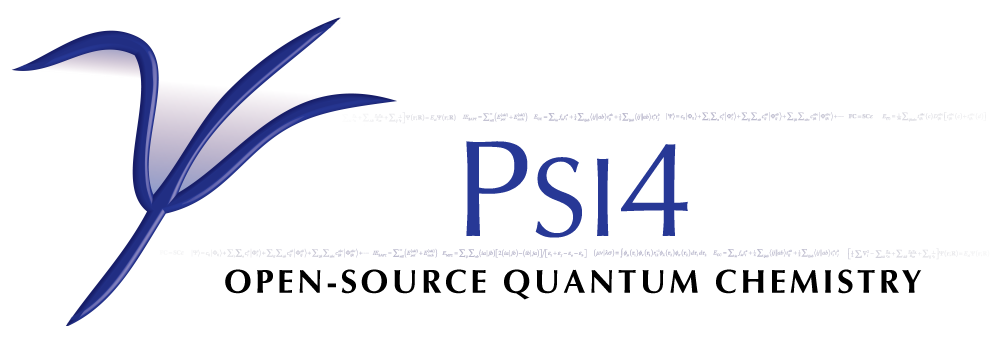# Complete Basis Set¶

Code author: Lori A. Burns

Section author: Lori A. Burns

complete_basis_set(name[, scf_basis, scf_scheme, corl_wfn, corl_basis, corl_scheme, delta_wfn, delta_wfn_lesser, delta_basis, delta_scheme, delta2_wfn, delta2_wfn_lesser, delta2_basis, delta2_scheme, delta3_wfn, delta3_wfn_lesser, delta3_basis, delta3_scheme, delta4_wfn, delta4_wfn_lesser, delta4_basis, delta4_scheme, delta5_wfn, delta5_wfn_lesser, delta5_basis, delta5_scheme])[source]

Function to define a multistage energy method from combinations of basis set extrapolations and delta corrections and condense the components into a minimum number of calculations.

Aliases: cbs() (float) – Total electronic energy in Hartrees

Caution

Some features are not yet implemented. Buy a developer a coffee.

• No way to tell function to boost fitting basis size for all calculations.
• No way to extrapolate def2 family basis sets
• Need to add more extrapolation schemes

As represented in the equation below, a CBS energy method is defined in several sequential stages (scf, corl, delta, delta2, delta3, delta4, delta5) covering treatment of the reference total energy, the correlation energy, a delta correction to the correlation energy, and a second delta correction, etc.. Each is activated by its stage_wfn keyword and is only allowed if all preceding stages are active.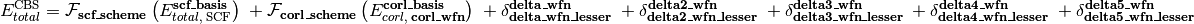Here,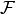is an energy or energy extrapolation scheme, and the following also hold.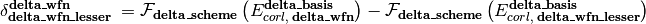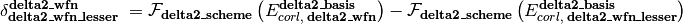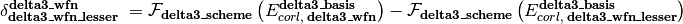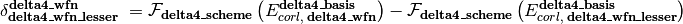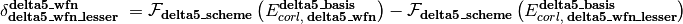A translation of this ungainly equation to example  below is as follows. In words, this is a double- and triple-zeta 2-point Helgaker-extrapolated CCSD(T) coupled-cluster correlation correction appended to a triple- and quadruple-zeta 2-point Helgaker-extrapolated MP2 correlation energy appended to a SCF/aug-cc-pVQZ reference energy.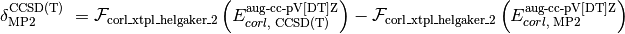• Energy Methods

The presence of a stage_wfn keyword is the indicator to incorporate (and check for stage_basis and stage_scheme keywords) and compute that stage in defining the CBS energy.

The cbs() function requires, at a minimum, name='scf' and scf_basis keywords to be specified for reference-step only jobs and name and corl_basis keywords for correlated jobs.

The following energy methods have been set up for cbs().

 scf mp2 mp2.5 mp3 mp4(sdq) mp4 mpn omp2 omp2.5 omp3 olccd lccd lccsd cepa(0) cepa(1) cepa(3) acpf aqcc qcisd cc2 ccsd fno-ccsd bccd cc3 qcisd(t) ccsd(t) fno-ccsd(t) bccd(t) cisd cisdt cisdtq cin fci mrccsd mrccsd(t) mrccsdt mrccsdt(q)
Parameters: name (string) – 'scf' || 'ccsd' || etc. First argument, usually unlabeled. Indicates the computational method for the correlation energy, unless only reference step to be performed, in which case should be 'scf'. Overruled if stage_wfn keywords supplied. scf_wfn (string) –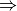'scf'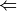|| 'c4-scf' || etc. Indicates the energy method for which the reference energy is to be obtained. Generally unnecessary, as ‘scf’ is the scf in Psi4 but can be used to direct lone scf components to run in Psi4 or Cfour in a mixed-program composite method. corl_wfn (string) – 'mp2' || 'ccsd(t)' || etc. Indicates the energy method for which the correlation energy is to be obtained. Can also be specified with name or as the unlabeled first argument to the function. delta_wfn (string) – 'ccsd' || 'ccsd(t)' || etc. Indicates the (superior) energy method for which a delta correction to the correlation energy is to be obtained. delta_wfn_lesser (string) –corl_wfn|| 'mp2' || etc. Indicates the inferior energy method for which a delta correction to the correlation energy is to be obtained. delta2_wfn (string) – 'ccsd' || 'ccsd(t)' || etc. Indicates the (superior) energy method for which a second delta correction to the correlation energy is to be obtained. delta2_wfn_lesser (string) –delta_wfn|| 'ccsd(t)' || etc. Indicates the inferior energy method for which a second delta correction to the correlation energy is to be obtained. delta3_wfn (string) – 'ccsd' || 'ccsd(t)' || etc. Indicates the (superior) energy method for which a third delta correction to the correlation energy is to be obtained. delta3_wfn_lesser (string) –delta2_wfn|| 'ccsd(t)' || etc. Indicates the inferior energy method for which a third delta correction to the correlation energy is to be obtained. delta4_wfn (string) – 'ccsd' || 'ccsd(t)' || etc. Indicates the (superior) energy method for which a fourth delta correction to the correlation energy is to be obtained. delta4_wfn_lesser (string) –delta3_wfn|| 'ccsd(t)' || etc. Indicates the inferior energy method for which a fourth delta correction to the correlation energy is to be obtained. delta5_wfn (string) – 'ccsd' || 'ccsd(t)' || etc. Indicates the (superior) energy method for which a fifth delta correction to the correlation energy is to be obtained. delta5_wfn_lesser (string) –delta4_wfn|| 'ccsd(t)' || etc. Indicates the inferior energy method for which a fifth delta correction to the correlation energy is to be obtained.
• Basis Sets

Currently, the basis set set through set commands have no influence on a cbs calculation.

Parameters: scf_basis (basis string) –corl_basis|| 'cc-pV[TQ]Z' || 'jun-cc-pv[tq5]z' || '6-31G*' || etc. Indicates the sequence of basis sets employed for the reference energy. If any correlation method is specified, scf_basis can default to corl_basis. corl_basis (basis string) – 'cc-pV[TQ]Z' || 'jun-cc-pv[tq5]z' || '6-31G*' || etc. Indicates the sequence of basis sets employed for the correlation energy. delta_basis (basis string) – 'cc-pV[TQ]Z' || 'jun-cc-pv[tq5]z' || '6-31G*' || etc. Indicates the sequence of basis sets employed for the delta correction to the correlation energy. delta2_basis (basis string) – 'cc-pV[TQ]Z' || 'jun-cc-pv[tq5]z' || '6-31G*' || etc. Indicates the sequence of basis sets employed for the second delta correction to the correlation energy. delta3_basis (basis string) – 'cc-pV[TQ]Z' || 'jun-cc-pv[tq5]z' || '6-31G*' || etc. Indicates the sequence of basis sets employed for the third delta correction to the correlation energy. delta4_basis (basis string) – 'cc-pV[TQ]Z' || 'jun-cc-pv[tq5]z' || '6-31G*' || etc. Indicates the sequence of basis sets employed for the fourth delta correction to the correlation energy. delta5_basis (basis string) – 'cc-pV[TQ]Z' || 'jun-cc-pv[tq5]z' || '6-31G*' || etc. Indicates the sequence of basis sets employed for the fifth delta correction to the correlation energy.
• Schemes

Transformations of the energy through basis set extrapolation for each stage of the CBS definition. A complaint is generated if number of basis sets in stage_basis does not exactly satisfy requirements of stage_scheme. An exception is the default, 'xtpl_highest_1', which uses the best basis set available. See Extrapolation Schemes for all available schemes.

Parameters: scf_scheme (function) –xtpl_highest_1|| scf_xtpl_helgaker_3 || etc. Indicates the basis set extrapolation scheme to be applied to the reference energy. Defaults to scf_xtpl_helgaker_3() if three valid basis sets present in scf_basis, scf_xtpl_helgaker_2() if two valid basis sets present in scf_basis, and xtpl_highest_1() otherwise. corl_scheme (function) –xtpl_highest_1|| corl_xtpl_helgaker_2 || etc. Indicates the basis set extrapolation scheme to be applied to the correlation energy. Defaults to corl_xtpl_helgaker_2() if two valid basis sets present in corl_basis and xtpl_highest_1() otherwise. delta_scheme (function) –xtpl_highest_1|| corl_xtpl_helgaker_2 || etc. Indicates the basis set extrapolation scheme to be applied to the delta correction to the correlation energy. Defaults to corl_xtpl_helgaker_2() if two valid basis sets present in delta_basis and xtpl_highest_1() otherwise. delta2_scheme (function) –xtpl_highest_1|| corl_xtpl_helgaker_2 || etc. Indicates the basis set extrapolation scheme to be applied to the second delta correction to the correlation energy. Defaults to corl_xtpl_helgaker_2() if two valid basis sets present in delta2_basis and xtpl_highest_1() otherwise. delta3_scheme (function) –xtpl_highest_1|| corl_xtpl_helgaker_2 || etc. Indicates the basis set extrapolation scheme to be applied to the third delta correction to the correlation energy. Defaults to corl_xtpl_helgaker_2() if two valid basis sets present in delta3_basis and xtpl_highest_1() otherwise. delta4_scheme (function) –xtpl_highest_1|| corl_xtpl_helgaker_2 || etc. Indicates the basis set extrapolation scheme to be applied to the fourth delta correction to the correlation energy. Defaults to corl_xtpl_helgaker_2() if two valid basis sets present in delta4_basis and xtpl_highest_1() otherwise. delta5_scheme (function) –xtpl_highest_1|| corl_xtpl_helgaker_2 || etc. Indicates the basis set extrapolation scheme to be applied to the fifth delta correction to the correlation energy. Defaults to corl_xtpl_helgaker_2() if two valid basis sets present in delta5_basis and xtpl_highest_1() otherwise. molecule (molecule) – h2o || etc. The target molecule, if not the last molecule defined.
>>> #  replicates with cbs() the simple model chemistry scf/cc-pVDZ: set basis cc-pVDZ energy('scf')
>>> cbs(name='scf', scf_basis='cc-pVDZ')

>>> #  replicates with cbs() the simple model chemistry mp2/jun-cc-pVDZ: set basis jun-cc-pVDZ energy('mp2')
>>> cbs(name='mp2', corl_basis='jun-cc-pVDZ')

>>> #  DTQ-zeta extrapolated scf reference energy
>>> cbs(name='scf', scf_basis='cc-pV[DTQ]Z', scf_scheme=scf_xtpl_helgaker_3)

>>> #  DT-zeta extrapolated mp2 correlation energy atop a T-zeta reference
>>> cbs(corl_wfn='mp2', corl_basis='cc-pv[dt]z', corl_scheme=corl_xtpl_helgaker_2)

>>> #  a DT-zeta extrapolated coupled-cluster correction atop a TQ-zeta extrapolated mp2 correlation energy atop a Q-zeta reference (both equivalent)
>>> cbs(corl_wfn='mp2', corl_basis='aug-cc-pv[tq]z', delta_wfn='ccsd(t)', delta_basis='aug-cc-pv[dt]z')
>>> cbs(energy, wfn='mp2', corl_basis='aug-cc-pv[tq]z', corl_scheme=corl_xtpl_helgaker_2, delta_wfn='ccsd(t)', delta_basis='aug-cc-pv[dt]z', delta_scheme=corl_xtpl_helgaker_2)

>>> #  a D-zeta ccsd(t) correction atop a DT-zeta extrapolated ccsd cluster correction atop a TQ-zeta extrapolated mp2 correlation energy atop a Q-zeta reference
>>> cbs(name='mp2', corl_basis='aug-cc-pv[tq]z', corl_scheme=corl_xtpl_helgaker_2, delta_wfn='ccsd', delta_basis='aug-cc-pv[dt]z', delta_scheme=corl_xtpl_helgaker_2, delta2_wfn='ccsd(t)', delta2_wfn_lesser='ccsd', delta2_basis='aug-cc-pvdz')

>>> #  cbs() coupled with database()
>>> TODO database('mp2', 'BASIC', subset=['h2o','nh3'], symm='on', func=cbs, corl_basis='cc-pV[tq]z', corl_scheme=corl_xtpl_helgaker_2, delta_wfn='ccsd(t)', delta_basis='sto-3g')

>>> #  cbs() coupled with optimize()
>>> TODO optimize('mp2', corl_basis='cc-pV[DT]Z', corl_scheme=corl_xtpl_helgaker_2, func=cbs)


Note

Presently (May 2016), only two of the five delta possibilities are active. Also, temporarily extrapolations are performed on differences of target and scf total energies, rather than on correlation energies directly. This doesn’t affect the extrapolated values of the particular formulas defined here (though it does affect the betas, which are commented out), but it is sloppy and temporary and could affect any user-defined corl extrapolations.

## Output¶

At the beginning of a cbs() job is printed a listing of the individual energy calculations which will be performed. The output snippet below is from the example job  above. It shows first each model chemistry needed to compute the aggregate model chemistry requested through cbs(). Then, since, for example, an energy('ccsd(t)') yields CCSD(T), CCSD, MP2, and SCF energy values, the wrapper condenses this task list into the second list of minimum number of calculations which will actually be run.

Naive listing of computations required.
scf / aug-cc-pvqz              for  SCF TOTAL ENERGY
mp2 / aug-cc-pvtz              for  MP2 CORRELATION ENERGY
mp2 / aug-cc-pvqz              for  MP2 CORRELATION ENERGY
ccsd(t) / aug-cc-pvdz              for  CCSD(T) CORRELATION ENERGY
ccsd(t) / aug-cc-pvtz              for  CCSD(T) CORRELATION ENERGY
mp2 / aug-cc-pvdz              for  MP2 CORRELATION ENERGY
mp2 / aug-cc-pvtz              for  MP2 CORRELATION ENERGY

Enlightened listing of computations required.
mp2 / aug-cc-pvqz              for  MP2 CORRELATION ENERGY
ccsd(t) / aug-cc-pvdz              for  CCSD(T) CORRELATION ENERGY
ccsd(t) / aug-cc-pvtz              for  CCSD(T) CORRELATION ENERGY


At the end of a cbs() job is printed a summary section like the one below. First, in the components section, are listed the results for each model chemistry available, whether required for the cbs job (*) or not. Next, in the stages section, are listed the results for each extrapolation. The energies of this section must be dotted with the weightings in column Wt to get the total cbs energy. Finally, in the CBS section, are listed the results for each stage of the cbs procedure. The stage energies of this section sum outright to the total cbs energy.

==> Components <==

----------------------------------------------------------------------------------
Method / Basis            Rqd   Energy [H]   Variable
----------------------------------------------------------------------------------
scf / aug-cc-pvqz        *  -1.11916375   SCF TOTAL ENERGY
mp2 / aug-cc-pvqz        *  -0.03407997   MP2 CORRELATION ENERGY
scf / aug-cc-pvdz           -1.11662884   SCF TOTAL ENERGY
mp2 / aug-cc-pvdz        *  -0.02881480   MP2 CORRELATION ENERGY
ccsd(t) / aug-cc-pvdz        *  -0.03893812   CCSD(T) CORRELATION ENERGY
ccsd / aug-cc-pvdz           -0.03893812   CCSD CORRELATION ENERGY
scf / aug-cc-pvtz           -1.11881134   SCF TOTAL ENERGY
mp2 / aug-cc-pvtz        *  -0.03288936   MP2 CORRELATION ENERGY
ccsd(t) / aug-cc-pvtz        *  -0.04201004   CCSD(T) CORRELATION ENERGY
ccsd / aug-cc-pvtz           -0.04201004   CCSD CORRELATION ENERGY
----------------------------------------------------------------------------------

==> Stages <==

----------------------------------------------------------------------------------
Stage         Method / Basis             Wt   Energy [H]   Scheme
----------------------------------------------------------------------------------
scf            scf / aug-cc-pvqz        1  -1.11916375   highest_1
corl            mp2 / aug-cc-pv[tq]z     1  -0.03494879   corl_xtpl_helgaker_2
delta        ccsd(t) / aug-cc-pv[dt]z     1  -0.04330347   corl_xtpl_helgaker_2
delta            mp2 / aug-cc-pv[dt]z    -1  -0.03460497   corl_xtpl_helgaker_2
----------------------------------------------------------------------------------

==> CBS <==

----------------------------------------------------------------------------------
Stage         Method / Basis                  Energy [H]   Scheme
----------------------------------------------------------------------------------
scf            scf / aug-cc-pvqz           -1.11916375   highest_1
corl            mp2 / aug-cc-pv[tq]z        -0.03494879   corl_xtpl_helgaker_2
delta  ccsd(t) - mp2 / aug-cc-pv[dt]z        -0.00869851   corl_xtpl_helgaker_2
total            CBS                         -1.16281105
----------------------------------------------------------------------------------


## Extrapolation Schemes¶

xtpl_highest_1(functionname, zHI, valueHI, verbose=True)[source]

Scheme for total or correlation energies with a single basis or the highest zeta-level among an array of bases. Used by complete_basis_set().scf_xtpl_helgaker_2(functionname, zLO, valueLO, zHI, valueHI, verbose=True, alpha=1.63)[source]

Extrapolation scheme for reference energies with two adjacent zeta-level bases. Used by complete_basis_set().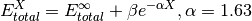scf_xtpl_helgaker_3(functionname, zLO, valueLO, zMD, valueMD, zHI, valueHI, verbose=True)[source]

Extrapolation scheme for reference energies with three adjacent zeta-level bases. Used by complete_basis_set().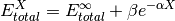corl_xtpl_helgaker_2(functionname, zLO, valueLO, zHI, valueHI, verbose=True)[source]

Extrapolation scheme for correlation energies with two adjacent zeta-level bases. Used by complete_basis_set().## Aliases¶

When a particular composite method or its functional form is going to be reused often, it is convenient to define an alias to it. A convenient place for such Python code to reside is in psi4/share/python/aliases.py (source location) or psi4/share/psi/python/aliases.py (installed location). No recompilation is necessary after defining an alias. Some existing examples are below.

sherrill_gold_standard(func, label, **kwargs)[source]

Function to call the quantum chemical method known as ‘Gold Standard’ in the Sherrill group. Uses complete_basis_set() to evaluate the following expression. Two-point extrapolation of the correlation energy performed according to corl_xtpl_helgaker_2().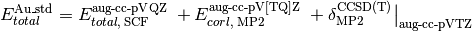>>> #  single-point energy by this composite method
>>> energy('sherrill_gold_standard')

>>> #  finite-difference geometry optimization
>>> optimize('sherrill_gold_standard')

>>> #  finite-difference geometry optimization, overwriting some pre-defined sherrill_gold_standard options
>>> optimize('sherrill_gold_standard', corl_basis='cc-pV[DT]Z', delta_basis='3-21g')

allen_focal_point(func, label, **kwargs)[source]

Function to call Wes Allen-style Focal Point Analysis. JCP 127 014306. Uses complete_basis_set() to evaluate the following expression. SCF employs a three-point extrapolation according to scf_xtpl_helgaker_3(). MP2, CCSD, and CCSD(T) employ two-point extrapolation performed according to corl_xtpl_helgaker_2(). CCSDT and CCSDT(Q) are plain deltas. This wrapper requires Kallay’s MRCC code.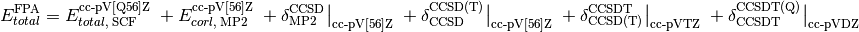>>> #  single-point energy by this composite method
>>> energy('allen_focal_point')

>>> #  finite-difference geometry optimization embarrasingly parallel
>>> optimize('allen_focal_point', mode='sow')